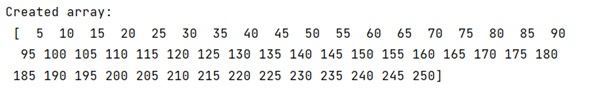# Is there a NumPy function that allows you to specify start, step, and number?

Learn about the function that allows you to specify start, step, and number in Python.
Submitted by Pranit Sharma, on February 19, 2023

NumPy is an abbreviated form of Numerical Python. It is used for different types of scientific operations in python. Numpy is a vast library in python which is used for almost every kind of scientific or mathematical operation. It is itself an array which is a collection of various methods and functions for processing the arrays.

## Underusing the function that allows you to specify start, step, and number

We know that numpy.linspace() is used to create an array using start, stop, and num whereas numpy.arange() is used to create an array using start, stop and step.

We need to find a way so we can blend the functionalities of linspace and arange so that we can create an array using start, step, and num.

For this purpose, we will define the start, step and num before creating the array and then numpy.arange() will help us.

We will create a numpy array using numpy.arange() where will pass the num value and multiply the result with the sum of the start and step.

Let us understand with the help of an example,

## Python code to demonstrate the NumPy function that allows you to specify start, step, and number

```# Import numpy
import numpy as np

# Defining start, step and num
start=5
step=5
num=50

# Creating an array
arr = np.arange(0,num)*step+start

# Display result
print("Created array:\n",arr)
```

Output: